• ## 知识表示学习模型

万次阅读 2019-10-01 13:43:34
写在前面 最近清华的THUNLP整理了Pre...本来这篇是打算写一写Knowledge Graph + BERT系列工作的，但是最近有在做知识图谱的一些东西所以就先整理一下知识表示学习(knowledge representation learning)的相关模型发展...
最近清华的THUNLP整理了Pre-trained Languge Model (PLM)相关的工作：PLMpapers，非常全面，想要了解最新NLP发展的同学不要错过。本来这篇是打算写一写Knowledge Graph + BERT系列工作的，但是最近有在做知识图谱的一些东西所以就先整理一下**知识表示学习(knowledge representation learning)**的相关模型发展，这样我们看后面的paper也会得心应手一些。1. A glance at Knowledge Representation Learning
What
首先什么是表示学习？其实我们早就在频繁使用了，比如词向量embedding，这种将研究对象的语义信息表示为稠密低维的实值向量的形式就叫表示学习。
有了表示学习的定义，那么知识表示学习(KRL) 就是面向知识库中实体和关系的表示学习，通过将实体或关系投影到低维向量空间，能够实现对实体和关系的语义信息的表示，可以高效地计算实体、关系及其之间的复杂语义关联。
Why
那么为什么会冒出知识表示学习呢？

显著提高计算效率。 简单地基于图算法计算实体间的语义和推理关系，其计算复杂度高、可扩展性差；而表示学习得到的分布式表示，则能够高效地实现语义相似度计算等操作。
有效缓解数据稀疏。 一方面将每个对象投影到低维的稠密向量空间；另一方面在投影过程中也可以借用高频对象的语义信息帮助低频对象的语义表示，捕获长尾分布。
实现异质信息融合。 将不同来源的对象投影到同一语义空间中，就能够建立统一的表示空间。

How
接下去会介绍知识表示学习的主要方法及前沿进展，这也是本系列博文的重要。首先给出整体定义：

知识库表示为 G=(E, R, S)，其中$E={\{e_{1},e_{2}, \cdots, e_{E}\}}$是实体集合，$R=\left\{r_{1}, r_{2}, \cdots, r_{R}\right\}$是关系集合，S是三元组集合，表示为(h, r, t)

基于距离的模型
基于翻译的模型
语义匹配模型
融合多源信息的模型
前沿进展

paper list ref

东南大学《知识图谱》课程

2. Distance-Based Models
UM Joint Learning of Words and Meaning Representations for Open-Text Semantic Parsing(2012)SELearning Structured Embeddings of Knowledge Bases(AAAI/2011)
在本文中，作者指出目前已有的知识库都是基于不同的框架，因此很难将它们整合应用到一个新的系统中。但是知识库中存在大量的结构化和组织化的数据，如果能够充分利用起来将会对AI领域非常有帮助。于是作者们提出一种模型将任何知识库中的实体和关系嵌入到一个更灵活的连续向量空间。嵌入可以认为是一个神经网络，该神经网络的特殊结构允许将原始数据结构集成到所学习的表示中。更准确地说，考虑到知识库是由一组实体和它们之间的关系定义的，该模型为每个实体（即一个低维向量）学习一个嵌入(embedding)，为每个关系（即矩阵）学习一个运算符(operator)。另外，在低维embedding空间使用核密度估计可以估量空间的概率密度，这样可以量化实体之间的关系存在可能性。
结构表示（Structured Embeddings，SE）中每个实体用$d$维的向量表示，所有实体被投影到同一个$d$维向量空间中。同时，SE还为每个关系定义了两个矩阵$\boldsymbol{M}_{r, 1}, \boldsymbol{M}_{r, 2} \in \mathbb{R}^{d \times d}$用于三元组中头实体和尾实体的投影操作。最后SE为每个三元组$(h, r, t)$定义了损失函数：
$f_{r}(h, t)=\left|\boldsymbol{M}_{r, 1} \boldsymbol{l}_{h}-\boldsymbol{M}_{r, 2} \boldsymbol{l}_{t}\right|_{L_{1}}$对于上述损失函数，我们可以理解为通过两个关系矩阵将头尾两个实体投影到同一关系空间中，然后在该空间中计算两投影向量的距离。而这个距离则反映了头尾实体之间在特定关系下的语义相似度，他们的距离越小则表明越有可能存在这种关系。
SE模型要学习的参数是实体向量矩阵$E$ 和两个关系矩阵$R^{lhs}$、$R^{rhs}$，下面是模型的训练过程：可以看出SE模型对头尾两个实体使用不同的矩阵进行投影，协同性较差，往往无法精确刻画两实体之间的语义联系。

3.  Trans-Based Models
TransE Translating Embeddings for Modeling Multi-relational Data(NIPS2013)
TransE是Trans系列模型的开山之作，也是知识图谱向量表示的baseline模型，后面会介绍它的很多变体。其实TransE的思想很简单，为了将实体与关系嵌入到低维的向量空间，对于知识库中的每个三元组$(h, r, t)$，认为头实体的embedding加上关系的embedding之后应该是与尾实体的embedding非常接近的。于是，可以将关系看做是两个实体之间的某种平移向量，如下图：按照上面的思路，即有了TransE模型的损失函数：$\mathcal{L}=\sum_{(h, \ell, t) \in S\left(h^{\prime}, \ell, t^{\prime}\right) \in S_{(h, \ell, t)}^{\prime}}\left[\gamma+d(\boldsymbol{h}+\ell, \boldsymbol{t})-d\left(\boldsymbol{h}^{\prime}+\ell, \boldsymbol{t}^{\prime}\right)\right]_{+}$这个损失函数是带有negative sampling和margin-based loss的

negative sampling：$S_{(h, \ell, t)}^{\prime}=\left\{\left(h^{\prime}, \ell, t\right) | h^{\prime} \in E\right\} \cup\left\{\left(h, \ell, t^{\prime}\right) | t^{\prime} \in E\right\}$表示负样本三元组，构造的方法是将S中每个三元组的头实体、关系和尾实体其中之一随机替换成其他的实体或关系，注意每个三元组只能同时替换一个元素。
margin-based loss：为了增强知识表示的区分能力，采用了最大间隔的方法，可以类比SVR的损失函数。$d(\boldsymbol{h}+\ell, \boldsymbol{t})$表示正样本的不相似得分，$d\left(\boldsymbol{h}^{\prime}+\ell, \boldsymbol{t}^{\prime}\right)$表示负样本的不相识得分，最小化这个损失函数可以使得正样本的得分越来越高而负样本的得分越来越低，并且只有两个得分差距大于某一阈值的时候loss才为0。

最终模型整体的训练过程如下：虽然TransE简单有效，但仍然存在一个非常严重的问题：不能很好处理复杂关系。举个栗子，有两个三元组(美国，总统，奥巴马)和(美国， 总统， 布什)，使用TransE模型表示的话会得到奥巴马和布什的表示向量非常接近，甚至完全相同。Code Here

TransHKnowledge Graph Embedding by Translating on Hyperplanes(AAAI2014)
相对于之前的知识表示模型，TransE有了很大的改进，但是当面对自反关系、一对多、多对一以及多对多这类复杂的关系时，就很难处理好（会使得一些不同的实体具有相同或者相近的向量表示）。于是作者提出TransH模型，在保证模型complexity和efficiency的同时，解决上述复杂关系的表示。
模型
TransH模型的主要思想是对每一个关系定义两个向量：超平面$w_{r}$和关系向量$d_{r}$。对于任意一个三元组$(h, r, t)$，$h$和$t$在超平面上的投影分别为$h_{\perp}$和$t_{\perp}$，如果是golden triplet，则存在$h_{\perp}+d_{r} \approx t_{\perp}$。看下图可能会更清楚一些：损失函数与TransE的一样：$\mathcal{L}=\sum_{(h, r, t) \in \Delta\left(h^{\prime}, r^{\prime}, t^{\prime}\right) \in \Delta_{(h, r, t)}^{\prime}}\left[f_{r}(\mathbf{h}, \mathbf{t})+\gamma-f_{r^{\prime}}\left(\mathbf{h}^{\prime}, \mathbf{t}^{\prime}\right)\right]_{+}$$f_{r}(\mathbf{h}, \mathbf{t})=\left\|\left(\mathbf{h}-\mathbf{w}_{r}^{\top} \mathbf{h} \mathbf{w}_{r}\right)+\mathbf{d}_{r}-\left(\mathbf{t}-\mathbf{w}_{r}^{\top} \mathbf{t} \mathbf{w}_{r}\right)\right\|_{2}^{2}$$\mathbf{h}_{\perp}=\mathbf{h}-\mathbf{w}_{r}^{\top} \mathbf{h} \mathbf{w}_{r}, \quad \mathbf{t}_{\perp}=\mathbf{t}-\mathbf{w}_{r}^{\top} \mathbf{t} \mathbf{w}_{r}$
模型整体训练过程也同TransE一样，这里就不再赘述。
负采样策略
不同于TransE模型中的随机替换头实体和尾实体之一作为负样本的方法，在TransH中指出：

However, as a real knowledge graph is often far from completed, this way of randomly sampling may introduce many false negative labels into training.

于是，在替换头实体或者尾实体的时候考虑了一个采样概率，$\frac{t p h}{t p h+h p t}$的概率替换头实体，$\frac{h p t}{t p h+h p t}$的概率替换尾实体，其中$tph$为每个头实体对应尾实体的平均数量，$hpt$为每个尾实体对应头实体的平均数量。也就是说，对于一对多的关系，更大的概率替换头实体；对于多对一的关系，更大概率替换尾实体。
Code Here

TransR Learning Entity and Relation Embeddings for Knowledge Graph Completion(AAAI2015)
前面介绍的TransE和TransH都是假设三元组的实体和关系在同一语义空间中的，尽管在TransH中采用了关系超平面的策略，但由于实体和关系是完全不同的，每一个实体可能会有多个aspect，即是是同一个实体在不同的关系下关注的可能是完全不相关的aspect，因此之前的模型还是不足以准确地表示knowledge graph。
于是，在本文中作者提出一种新模型TransR，其思想就是将实体和关系的表示空间区分开来，实体有entity space，关系有relation space，在计算时通过一个投射矩阵$M_{r}$将实体映射到关系空间进行计算。
$投影过程：\mathbf{h}_{r}=\mathbf{h} \mathbf{M}_{r}, \quad \mathbf{t}_{r}=\mathbf{t} \mathbf{M}_{r}$$打分函数： f_{r}(h, t)=\left\|\mathbf{h}_{r}+\mathbf{r}-\mathbf{t}_{r}\right\|_{2}^{2}$$损失函数：L=\sum_{(h, r, t) \in S\left(h^{\prime}, r, t^{\prime}\right) \in S^{\prime}} \max \left(0, f_{r}(h, t)+\gamma-f_{r}\left(h^{\prime}, t^{\prime}\right)\right)$此外，不仅仅实体有多个aspect，对于关系，也可能存在多义性，比如关系（location location contains）其实包含country-city、country-university、continent-country等多种含义。为此，作者们提出CTransR，即Cluster-based TransR，对同一关系下的不同实体对进行聚类并学习到关系在不同聚类簇的表示。具体做法是

利用TransE训练$(h, r, t)$三元组
利用vector offset $(h-t)$进行聚类
对每一类的子关系都学习到一个变换矩阵$M_{r}$和表示向量$r_{c}$
$f_{r}(h, t)=\left\|\mathbf{h}_{r, c}+\mathbf{r}_{c}-\mathbf{t}_{r, c}\right\|_{2}^{2}+\alpha\left\|\mathbf{r}_{c}-\mathbf{r}\right\|_{2}^{2}$

Code Here

TransDKnowledge graph embedding via dynamic mapping matrix(ACL2015)
文章首先指出了TransR的三个问题：

在TransR中，对于特定关系，所有实体共享同一映射矩阵。但是实际情况头尾实体可能属于不同范畴，例如(美国，总统，特朗普)这个三元组中，美国和特朗普就不是相同范畴的实体，因此应该采用不同的映射矩阵；
投影运算是实体与关系的交互过程，映射矩阵仅由关系确定是不合理的；
矩阵向量乘法运算量大，当关系数大时，矩阵向量乘法的参数也比TransE和TransH多。

基于此，作者们提出一种基于动态映射矩阵的TransD模型。对于每个三元组$(h, r, t)$，其中每个元素都有两种向量表示，一种是构建动态映射矩阵向量$(h_{p}, r_{p}, t_{p})$；另外一种是用于自身实体/关系语义表示$(\bold h, \bold r, \bold t)$。首先是利用头尾实体的映射向量构建映射矩阵：\begin{aligned} \mathbf{M}_{r h} &=\mathbf{r}_{p} \mathbf{h}_{p}^{\top}+\mathbf{I}^{m \times n} \\ \mathbf{M}_{r t} &=\mathbf{r}_{p} \mathbf{t}_{p}^{\top}+\mathbf{I}^{m \times n} \end{aligned}接着通过各自的映射矩阵将实体投影到关系空间：$\mathbf{h}_{\perp}=\mathbf{M}_{r h} \mathbf{h}, \quad \mathbf{t}_{\perp}=\mathbf{M}_{r t} \mathbf{t}$整体的打分函数和损失函数以及训练过程都跟之前的模型一样。Code Here

TranSparse Knowledge Graph Completion with Adaptive Sparse Transfer Matrix(AAAI2016)
之前的Trans系列模型都忽略了知识表示面临的两个问题：

异构性(heterogeneous)：不同关系连接的实体对数量差别巨大
不平衡性(imbalanced)：同一关系链接的头实体和尾实体数量差别巨大，例如对于关系性别，头实体的数量很多（‘小明’‘小花’etc），而对于尾实体仅仅只有两个（‘男’‘女’）如果使用同一模型来处理所有情况的话，则可能由于异构性导致对于复杂关系（链接数量多）欠拟合，对简单关系（链接数量少）过拟合。为了解决异构性，作者提出TranSparse模型用自适应的稀疏矩阵代替一般的映射矩阵。
TranSparse(share)
为了解决异构性问题，TranSparse将transfer matrix设置为自适应的稀疏矩阵，对于复杂关系，我们需要更多的参数去学习其中包含的信息，所以transfer matrix的稀疏度会比较低，即有更多的元素不为0；而对于简单的关系则恰好相反。而这里稀疏度由$\theta_{r}$定义：
$\theta_{r}=1-\left(1-\theta_{\min }\right) N_{r} / N_{r^{*}}$其中，$\theta_{min}$是一个0-1之间的最小稀疏度超参，$N_{r}$表示关系$r$链接的实体对数量，$N_{r^{*}}$表示其中链接的最大值。
TranSparse(share)这里没有考虑不平衡性，所以对于头尾实体的mapping过程是一样的：$\mathbf{h}_{p}=\mathbf{M}_{r}\left(\theta_{r}\right) \mathbf{h}, \quad \mathbf{t}_{p}=\mathbf{M}_{r}\left(\theta_{r}\right) \mathbf{t}$
TranSparse(separate)
为了解决不平衡性问题，这里更细致地对头实体和尾实体设置了不同的transfer matrix：$\theta_{r}^{l}=1-\left(1-\theta_{\min }\right) N_{r}^{l} / N_{r^{*}}^{l^{*}} \quad(l=h, t)$$\mathbf{h}_{p}=\mathbf{M}_{r}^{h}\left(\theta_{r}^{h}\right) \mathbf{h}, \quad \mathbf{t}_{p}=\mathbf{M}_{r}^{t}\left(\theta_{r}^{t}\right) \mathbf{t}$
模型训练
两者（share和separate）的打分函数都是：$f_{r}(\mathbf{h}, \mathbf{t})=\left\|\mathbf{h}_{p}+\mathbf{r}-\mathbf{t}_{p}\right\|_{\ell_{1 / 2}}^{2}$$L=\sum_{(h, r, t) \in \Delta\left(h^{\prime}, r, t\right) \in \Delta^{\prime}}\left[\gamma+f_{r}(\mathbf{h}, \mathbf{t})-f_{r}\left(\mathbf{h}^{\prime}, \mathbf{t}^{\prime}\right)\right]_{+}$Code Here

TransM Transition-based knowledge graph embedding with relational mapping properties(2014)
TransM也是为了解决TransE只能处理一对一关系的问题，对于存在多种选择的一方给了更多的自由度，即$h+r \approx t$，如下图具体做法是在得分函数的前面加上了权重$w_{r}$
$w_{r}=\frac{1}{\log \left(h_{r} p t_{r}+t_{r} p h_{r}\right)}$其中$h_{r}pt_{r}$表示尾实体对应的头实体数量(heads per tail)，$t_{r}ph_{r}$同理。$打分函数： f_{r}(h, t)=w_{\mathbf{r}}\|\mathbf{h}+\mathbf{r}-\mathbf{t}\|_{L_{1} / L_{2}}$

ManiFoldE From One Point to A Manifold: Knowledge Graph Embedding For Precise Link Prediction(IJCAI2016)

ill-posed algebraic problem：指一个方程组中的方程式个数远大于变量个数，这会导致求得的解经常是不精确且不稳定的。之前的翻译模型都是基于$\mathbf{h}_{\mathbf{r}}+\mathbf{r}=\mathbf{t}_{\mathbf{r}}$，如果三元组的数量为$T$，embedding的维度为$d$， 则一共有$T*d$个方程，而所需要学习的变量一共有$(E+R)*D$个，由于$T>>(E+R)$，那么这类模型由于ill-posed algebraic problem无法给出精准链接预测；
adopting an overstrict geometric form：TransE过于严格的限制使得链接预测得到的基本都为一个点，这对于多对多关系显然是不正确的。虽然在之前有TransH， TransR等模型对此进行优化，但是将关系映射到另外一个子空间后仍然会存在该问题。

为了解决上述两个问题，作者提出一种基于流形的模型，将约束$\mathbf{h}_{\mathbf{r}}+\mathbf{r}=\mathbf{t}_{\mathbf{r}}$进行放宽$\mathcal{M}(\mathbf{h}, \mathbf{r}, \mathbf{t})=D_{r}^{2}$其中$\mathcal{M}$是流形函数$M(h, r, t)=\|h+r-t\|_{l 2}$
以$(h, r, *)$为例，所有合适的尾实体都分布在高维流形上，举个栗子，$\mathcal{M}$是一个高维球体，则所有的尾实体都在以$h+t$为球心，以$D_{r}$为半径的球面上。
$打分函数：f(h, r, t)=\left\|M(h, r, t)-D_{r}^{2}\right\|_{l 1 / 2}$
$目标函数：\mathcal{L}=\sum_{(h, r, t)} \sum_{\left(h^{\prime}, r^{\prime}, t^{\prime}\right) \in \Delta^{\prime}}\left[f_{r}^{\prime}\left(h^{\prime}, t^{\prime}\right)-f_{r}(h, t)+\gamma\right]_{+}$
Code Here

TransF Knowledge Graph Embedding by Flexible Translation(2016)
TransF提出主要是为了解决TransE的一对一关系问题，虽然在之前有TransH提出解决思路，但是引入了更多的参数以及更大的复杂度。于是TransF就是为了解决一对多多对一以及多对多关系，同时又不增加参数。具体就是将TransE的限制条件$\mathbf{h}+\mathbf{r} \approx \mathbf{t}$放宽为$\mathbf{h}+\mathbf{r} \approx \alpha \mathbf{t}$，如下图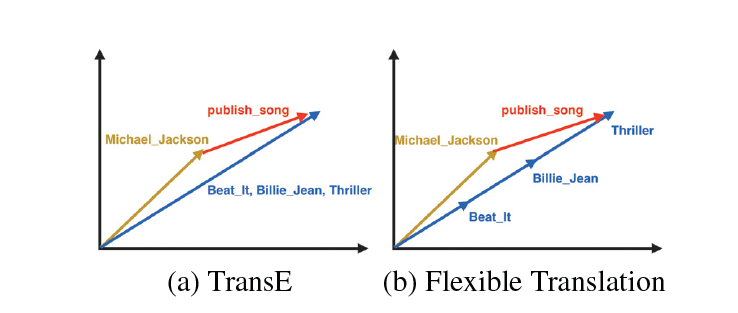得分函数同时衡量了$h+t$和$t$，$t-h$和$h$的方向：
$f(h, r, t)=(h+r)^{T} t+(t-r)^{T} h$

TransA TransA: An Adaptive Approach for Knowledge Graph Embedding(2015)
作者首先提出了两个TransE模型的不足：

loss metric：TransE的loss metric为$\|\mathbf{h}+\mathbf{r}-\mathbf{t}\|_{2}^{2}$，是一种欧氏距离的计算，其对应的图形是一个圆。由于这种简单的loss metric导致不能很好处理复杂的关系（如一对多、多对一等）。论文中给出一个详细案例，从下图可知蓝色部分为正例，红色部分为负例，TransE 模型划分错了七个点。而本文提出了一种基于马氏距离的 TransA 模型，其 PCA 降维图形对应的是一个椭圆，该模型只分错了三个点。identical dimension：TransE等之前的模型等价地对待向量中的每一维度，但是实际上各个维度的重要度是不相同的，有些维度是有作用的，有些维度却是噪音。还是以论文中的栗子，对于关系HasPart来说，左边的TransE模型根据欧氏距离计算得出(room, haspart, goniff)这样错误的三元组。通过对x，y轴进行分解之后发现，关系HasPart会更关注与x轴相近的实体。TransA模型为此引入了对每一维度的加权矩阵。模型
TransA模型对之前的打分函数进行了改进，引入加权矩阵$W_{r}$：$f_{r}(h, t)=(|\mathbf{h}+\mathbf{r}-\mathbf{t}|)^{\top} \mathbf{W}_{\mathbf{r}}(|\mathbf{h}+\mathbf{r}-\mathbf{t}|)$并通过LDL对$W_{r}$进行分解：$\mathbf{W}_{\mathbf{r}}=\mathbf{L}_{\mathbf{r}}^{\top} \mathbf{D}_{\mathbf{r}} \mathbf{L}_{\mathbf{r}}$整理后得到最终的打分函数：$f_{r}=\left(\mathbf{L}_{\mathbf{r}}|\mathbf{h}+\mathbf{r}-\mathbf{t}|\right)^{\top} \mathbf{D}_{\mathbf{r}}\left(\mathbf{L}_{\mathbf{r}}|\mathbf{h}+\mathbf{r}-\mathbf{t}|\right)$训练过程损失函数\begin{aligned} \min & \sum_{(h, r, t) \in \Delta\left(h^{\prime}, r^{\prime}, t^{\prime}\right) \in \Delta^{\prime}}\left[f_{r}(h, t)+\gamma-f_{r^{\prime}}\left(h^{\prime}, t^{\prime}\right)\right]_{+}+\\ & \lambda\left(\sum_{r \in R}\left\|\mathbf{W}_{\mathbf{r}}\right\|_{F}^{2}\right)+C\left(\sum_{e \in E}\|\mathbf{e}\|_{2}^{2}+\sum_{r \in R}\|\mathbf{r}\|_{2}^{2}\right) \\ \text { s.t. } &\left[\mathbf{W}_{\mathbf{r}}\right]_{i j} \geq 0 \end{aligned}

KG2E Learning to Represent Knowledge Graphs with Gaussian Embedding(2015)
作者指出TransE、TransR等之前的研究，在分离正三元组及其对应的负三元组时，不同的实体和关系往往共享相同的边界，而知识库中实体和关系的**（非）确定性**被完全忽略。但实际上，不同的实体和关系常常包含不同的确定性。在这里，一个实体/关系的（非）确定性表示在将一个三元组作为上下文评分时表示其语义的可信度。举个栗子，在推断一个人时，配偶关系的确定性明显大于国籍。在预测Hillary Clinton时，当我们知道她丈夫（配偶）是Bill Clinton时，我们可能更有信心知道她是谁，而不是知道她出生在（国籍）美国。
为此，本文使用Gaussian Distribution 来表示实体和关系，使用协方差来表示实体和关系的不确定度。相同颜色的圆圈代表同意三元组，圆圈大小则表示不同三元组的不确定度。相对于之前的point-based model，本文的KG2E是一种density-based model。在建模过程中强调了(un)certainty的概念，融入关系和实体语义本身的不确定性并使用高斯分布协方差表示实体关系的不确定度，使用高斯分布的均值表示实体或关系在语义空间中的中心值。

TransG TransG : A Generative Model for Knowledge Graph Embedding(2016)
本文主要是为了解决多关系语义（multiple relation semantics），即某一种关系可能会存在多个类别的语义，比如对于(Atlantics, HasPart, NewYorkBay)和(Table, HasPart, Leg)两个三元组都属于关系HasPart，但是他们在语义上并不相同，一个是位置的语义，一个是部件的语义。其实这个问题在之前介绍的CTransR中也提出过解决方案，但是作者似乎认为CTransR预处理聚类的操作不是很elegant。为此，作者们提出了一种基于贝叶斯非参的无限混和嵌入模型：认为关系向量由若干子成分向量合成，模型会根据实体对自动选择一个关系向量，而多少个这样的关系向量还可以由模型自动选择。 整体过程如下：一张总结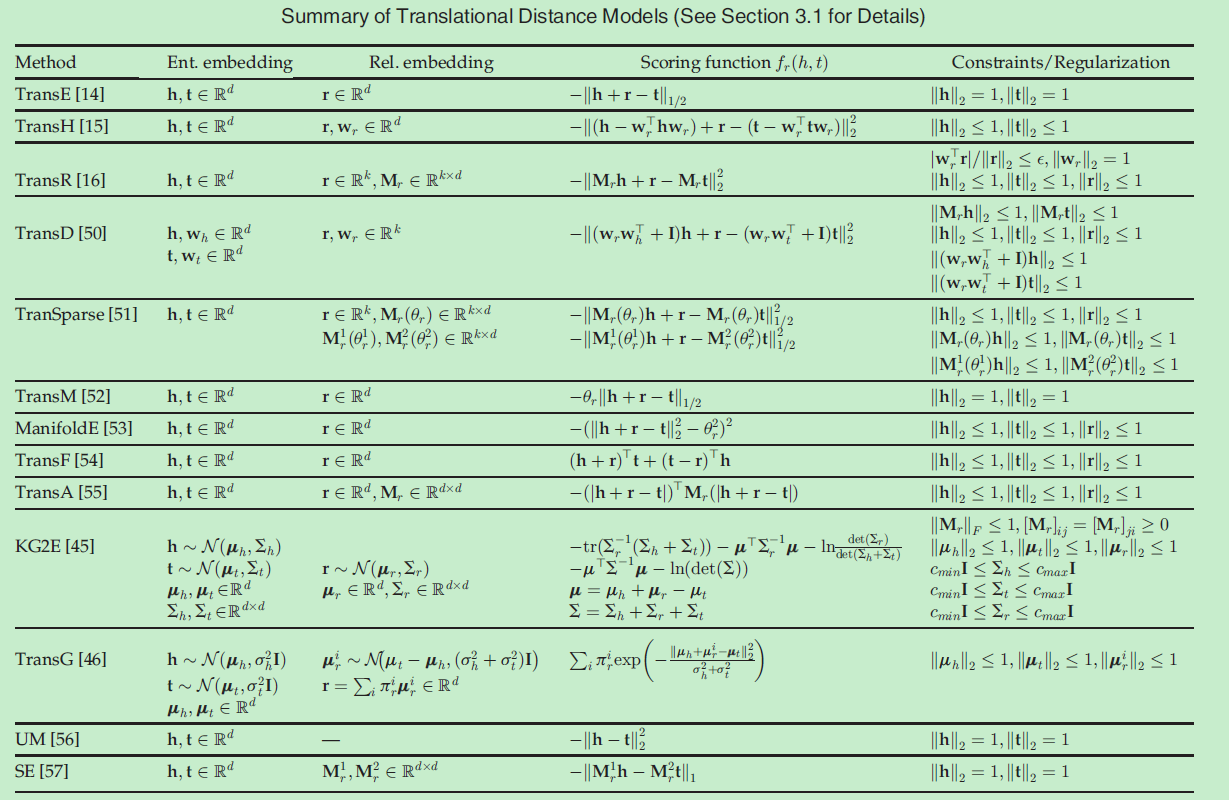以上
2019.10.08


展开全文Trans系列
• Paper: 刘知远 2016 知识表示学习研究进展 计算机研究与发展 1. 表示学习旨在将研究对象的语义信息表示为稠密低维表示向量；而知识表示学习则面向知识库中的实体和关系进行表示学习；该技术可以在低维空间中高效...
知识表示学习研究进展

本博文来源于 Paper: 刘知远 2016 知识表示学习研究进展 计算机研究与发展

目前的产品

谷歌知识图谱
微软Bing Satori
百度知心
搜狗知立方
IBM Waston等

知识库的主要研究目标

从无结构或半结构的互联网信息中获取有结构知识，自动融合构建知识库、服务知识推理等相关应用，自动融合构建知识库、服务知识推理等相关应用。知识表示是知识获取与应用的基础，因此，知识表示学习问题是贯穿知识库的构建与应用全过程的关键问题。

基于网络形式的知识表示面临的困难点

计算效率问题；
数据稀疏问题：大规模知识库遵循长尾分布；

表示学习 VS 知识表示学习

表示学习旨在将研究对象的语义信息表示为稠密低维表示向量；而知识表示学习则面向知识库中的实体和关系进行表示学习；该技术可以在低维空间中高效计算实体和关系的语义联系，有效解决数据稀疏问题，使知识获取、融合、推理的性能得到显著提升。

表示学习

表示学习旨在将研究对象的语义信息表示为稠密低维实值向量。在该低维向量空间中，2个对象距离越近则说明其语义相似度越高。通常将研究对象表示为向量：实体 e 表示为le${l}_{e}$$l_e$；关系 r 表示为 lr${l}_{r}$$l_r$；通过欧氏距离或余弦距离等方式计算任意2个对象之间的语义相似度；

独热表示 one-hot encoding

独热表示是信息检索和搜索引擎中广泛使用的词袋模型的基础。

词袋模型

一个网页中有W个不同的词，则在词袋模型中都被表示为一个W维的独热表示向量。在此基础上，词袋模型将每个文档表示为一个W维向量，每一位表示对应的额词在该文档的重要性。将研究对象表示为向量，该向量只有某一维非零，其他维度上的值均为0.有多少个不同的研究对象，独热表示向量就有多长。

优点：无学习过程，简单高效
缺点：假设所有对象都是相互独立的。导致无法有效表示短文本、容易受到数据稀疏问题影响的根本原因；

知识表示学习

知识表示学习是面向知识库中实体和关系的表示学习。通过将实体或关系投影到低维向量空间，我们能够实现对实体和关系的语义信息的表示，可以高效地计算实体、关系及其之间的复杂语义关联。

知识表示学习得到的分布式表示有以下典型应用：

相似度计算：计算实体键的语义相似度；
关系抽取；
自动问答；
实体链接；

知识表示学习的主要优点

显著提升计算效率。
知识库的三元组表示实际就是基于独热表示的。此方法，计算复杂度高、可扩展性差。
表示学习得到的分布式表示，能够高效地实现语义相似度计算等操作，显著提升计算效率。
有效缓解数据稀疏：
由于表示学习将对象投影到统一的低维空间中，使每个对象均对应一个稠密向量，从而缓解数据稀疏问题；体现在两个方面：
每个对象的向量均为稠密有值的，可能度量任意对象之间的语义相似程序；
将大量对象投影到统一空间的过程，能够将高频对象的语义信息用于帮助低频对象的语义表示，提高低频对象的语义表示的精确性；
实现异质信息融合；
不同来源的异质信息需要融合为整体，才能得到有效应用。
大量实体和关系在不同知识库中的名称不同。通过设计合理的表示学习模型，将不同来源的对象投影到同一个语义空间中，就能够建立统一的表示空间，实现多知识库的信息融合。

表示学习的几个代表模型

距离模型：结构表示（structured embedding, SE）
每个实体用d维向量表示，所有实体被投影到同一个d维向量空间中。
每个关系定义为2个矩阵Mr,1,Mr,2∈Rd×d${M}_{r,1},{M}_{r,2}\in {R}^{d×d}$$M_{r,1}, M_{r,2} \in R^{d \times d}$，用于三元组中头实体和尾实体的投影操作。
损失函数：fr(h,t)=|Mr,llh−Mr,2lt|L1${f}_{r}\left(h,t\right)=|{M}_{r,l}{l}_{h}-{M}_{r,2}{l}_{t}|{L}_{1}$$f_r (h, t) = \vert M_{r, l} l_h - M_{r, 2} l_t \vert L_1$
计算两投影向量的距离；
反映了2个实体在关系r下的语义相关度；
距离越小，说明2个实体存在这种关系的可能性越大。
学习样例：知识库三元组；
通过优化模型参数使知识库三元组的损失函数值不断降低，从而使实体向量和关系矩阵能够较好地反映实体和关系的语义信息。
链接预测：找两实体距离最近的关系矩阵
缺陷：它对头、尾实体使用2个不同的矩阵进行投影，协同性较差，往往无法精确刻画两实体与关系之间的语义联系。
单层神经网络模型（single layer model, SLM）：
采用非线性操作，来减轻SE无法协同精确刻画实体与关系的语义联系的问题。
SLM是SE模型的改进版本，但是它的非线性操作仅提供了实体和关系之间比较微弱的联系。于此同时，却引入了更高的计算复杂度。
能量模型：
语义匹配能量模型（semantic matching energy， SME）：寻找实体和关系之间的语义联系；
每个实体和关系都用低维向量表示；
定义若干投影矩阵，刻画实体与关系的内在联系；
SME定义2种评分函数：线性形式和双线性形式；
缺点：操作复杂；
双线性模型：
隐变量模型（latent factor model， LFM）
利用基于关系的双线性变换，刻画实体和关系之间的二阶联系。
双线性评分函数 fr(h,t)=lThMrlt${f}_{r}\left(h,t\right)={l}_{h}^{T}{M}_{r}{l}_{t}$$f_r (h, t) = l_h^T M_r l_t$
优点：通过简单有效的方法刻画了实体和关系的语义联系，协同性较好，计算复杂度低。
后来的DISTMULT模型简化了LFM模型，将关系矩阵设置为对角阵。不仅极大降低了模型复杂度，模型效果反而得到显著提升。
张量神经网络模型（Neural tensor network，NTN）：
基本思想：用双线性张量取代传统神经网络中的线性变换层，在不同维度下将头、尾实体向量联系起来；
NTN中的实体向量是该实体中所有单词向量的平均值。
好处：实体中的单词数量远小于实体数量，可以充分重复利用单词向量构建实体表示，降低实体表示学习的洗属性问题，增强不同实体的语义联系。
缺点：由于引入张量操作，虽然能够更精确地刻画实体和关系的复杂语义联系，但是计算复杂度非常高，需要大量三元组样例才能得到充分学习。NTN在大规模稀疏知识图谱上的效果较差。
矩阵分解模型：
矩阵分解是得到低维向量表示的重要途径。
代表方法：RESACL模型；
基本思想与LFM类似。不同之处在于，RESACL会优化张量中的所有位置，包括为0的位置；LFM只会优化知识库中存在的三元组；
翻译模型TransE：
起源于word2vec词表示学习模型，词向量空间存在有趣的平移不变现象；
平移不变现象普遍存在于词汇的语义关系和句法关系中；此现象产生了TransE模型；
TransE模型将知识库中的关系看做实体间的某种平移向量。用关系r的向量 lr${l}_{r}$$l_r$ 作为头实体向量 lh${l}_{h}$$l_h$ 和尾实体向量lt${l}_{t}$$l_t$ 之间的平移。也可以将 lr${l}_{r}$$l_r$ 看作从lh${l}_{h}$$l_h$ 到 lt${l}_{t}$$l_t$ 之间的翻译。
TransE希望 lh+lr∼lt${l}_{h}+{l}_{r}\sim {l}_{t}$$l_h + l_r \sim l_t$
损失函数：
fr(h,t)=|lh+lr−lt|L1/L2${f}_{r}\left(h,t\right)=|{l}_{h}+{l}_{r}-{l}_{t}{|}_{{L}_{1}/{L}_{2}}$$f_r (h, t) = \vert l_h + l_r - l_t \vert_{L_1 / L_2}$
上式即为 lh+lr${l}_{h}+{l}_{r}$$l_h + l_r$ 和 lt${l}_{t}$$l_t$ 的 L1或 L2距离。
为了增强知识表示的区分能力，TransE采用最大间隔方法
全息表示模型（holographic embeddings， Hole）:
使用头、尾实体向量的“循环相关”操作来表示该实体对。
循环相关操作可以看做张量乘法特殊形式，具有较强的表达能力，优点：
不可交换性；
相关性；
计算效率高；
该模型刚刚提出，尚未验证其效果，但是无疑为知识表示学习提供了全新的视角，值得关注。
TransE面临的3个主要难点：
难点一：复杂关系建模：
由于模型简单，在大规模知识图谱上效果明显。但是也由于过于简单，导致TransE在处理知识库的复杂关系时捉襟见肘；
将关系划分为：1-1；1-N；N-1；N-N；四种模型；
1-N；N-1；N-N；定义为复杂关系；
由于复杂关系的存在，TransE学习得到的实体表示区分性较低。
为了解决TransE存在的一些问题，衍生出一些模型。有8个代表模型:
TransH模型：
假设实体和关系处于相同的语义空间R^d 中，限制了其表示能力；
为了解决TransE模型在处理1-N；N-1；N-N；复杂关系时的局限性，TransH模型提出让一个实体在不同的关系下拥有不同的表示；
对于关系r, TransH模型同时使用平移向量lr${l}_{r}$$l_r$ 和超平面的法向量w_r 来表示它.对于一个三元组(h, r, t) , TransH首先将头实体向量lh${l}_{h}$$l_h$和尾实体向量lr${l}_{r}$$l_r$ 沿法线wr${w}_{r}$$w_r$ 投影到关系r对应的超平面上，用lhr${l}_{{h}_{r}}$$l_{h_r}$ 和ltr${l}_{{t}_{r}}$$l_{t_r}$ 表示如下：
lhr=lh−wTrlhwr${l}_{{h}_{r}}={l}_{h}-{w}_{r}^{T}{l}_{h}{w}_{r}$$l_{h_r} = l_h - w_r^T l_h w_r$
ltr=lr−wTrltwr${l}_{{t}_{r}}={l}_{r}-{w}_{r}^{T}{l}_{t}{w}_{r}$$l_{t_r} = l_r - w_r^T l_t w_r$
TransH 定义了如下损失函数：fr(h,t)=‖hhr+lr−ltr‖L1/l2${f}_{r}\left(h,t\right)=‖{h}_{{h}_{r}}+{l}_{r}-{l}_{{t}_{r}}{‖}_{{L}_{1}/{l}_{2}}$$f_r (h, t) = \Vert h_{h_r} + l_r - l_{t_r} \Vert_{L_1 / l_2}$
TransR | CTransR模型
虽然TransH模型使每个实体在不同关系下拥有了不同的表示，它仍然假设实体和关系处于相同的语义空间R^d 中，这一定程度上限制了TransH的表示能力.
TransR 认为，一个实体是多种属性的综合体，不同关系关注实体的不同属性；不同的关系拥有不同的语义空间。
对于每个三元组，首先应将实体投影到对应的关系空间中，然后在建立从头实体到尾实体的翻译关系；
TransR定义投 影矩阵将实体向量从实体空间投影到关系r的子空间，用lhr${l}_{{h}_{r}}$$l_{h_r}$ 和 ltr${l}_{{t}_{r}}$$l_{t_r}$ 表示如下：
lhr=lhMr${l}_{{h}_{r}}={l}_{h}{M}_{r}$$l_{h_r} = l_h M_r$
ltr=ltMr${l}_{{t}_{r}}={l}_{t}{M}_{r}$$l_{t_r} = l_t M_r$
然后使 lhr+lr≈ltr${l}_{{h}_{r}}+{l}_{r}\approx {l}_{{t}_{r}}$$l_{h_r} + l_r \approx l_{t_r}$. 因此，TransR定义了如下损失函数：fr(h,t)=‖lhr+lr−ltr‖L1/L−2${f}_{r}\left(h,t\right)=‖{l}_{{h}_{r}}+{l}_{r}-{l}_{{t}_{r}}{‖}_{{L}_{1}/L-2}$$f_r (h, t) = \Vert l_{h_r} + l_r - l_{t_r} \Vert_{L_1 / L-2}$
TransR缺点：
在同一个关系r下，头、尾实体共享相同的投影矩阵，然而，一个关系的头、尾实体的类型或属性可能差异巨大。
从实体空间到关系空间的投影是实体和关系之间的交互过程，因此，TransR让投影矩阵仅与关系有关是不合理的；
相比于TransE和TransH，TransR由于引入了空间投影，使得TransR模型参数急剧增加，计算复杂度大大提高；
CTransE模型
通过将把关系 r 对应的实体对的向量差值 lh−lt${l}_{h}-{l}_{t}$$l_h - l_t$ 进行聚类，将关系r细分为多个子关系 rc${r}_{c}$$r_c$.
为每一个子关系 rc${r}_{c}$$r_c$ 分别学习向量表示；
对于每个三元组（h, r, t），定义了损失函数：fr(h,t)=‖lhr+lrc−ltr‖L1/L2${f}_{r}\left(h,t\right)=‖{l}_{{h}_{r}}+{l}_{{r}_{c}}-{l}_{{t}_{r}}{‖}_{{L}_{1}/{L}_{2}}$$f_r (h, t) = \Vert l_{h_r} + l_{r_c} - l_{t_r} \Vert_{L_1 / L_2}$
TransD模型
为了解决TransR模型中存在的问题；
设置2个分别将实体和尾实体投影到关系空间的投影矩阵 Mrh${M}_{r}h$$M_rh$和 Mrt${M}_{r}t$$M_rt$
Mrh${M}_{r}h$$M_rh$和 Mrt${M}_{r}t$$M_rt$ 与实体和关系均相关；
利用2个投影向量构建投影矩阵，解决了原来TransR模型参数过多的问题。
TranSparse模型
知识库中的实体和关系的异质性和不平衡性是制约知识表示学习的难题：
异质性：知识库中某些关系可能会与大量的实体有连接，而某些关系则可能仅仅与少量实体有连接；
不均衡性：某些关系中，头实体和尾实体的种类和数量可能差别巨大；
TranSparse提出使用稀疏矩阵代替TransR模型中的稠密矩阵，其中矩阵M_r 的稀疏度由关系r连接的实体对数量决定。
为了解决关系的不平衡问题，TranSparse对于头实体和尾实体分别使用2个不同的投影矩阵
TransA模型
TransE及其之后的扩展模型均存在2个重要问题：
损失函数只采用 L1${L}_{1}$$L_1$ 或 L2${L}_{2}$$L_2$ 距离，灵活性不够；
损失函数过于简单，实体和关系向量的每一维等同考虑；
改进：将损失函数中的距离度量改用马氏距离，并为每一维学习不同的权重。
TransG模型：
使用高斯混合模型描述头、尾实体之间的关系；
一个关系会应对多种语义，每种语义用一个高斯分布来刻画。
通过考虑关系r的不同语义，形成多个高斯分布，就能够区分出正确和错误实体；
KG2E模型
认为，知识库中关系和实体的语义本身具有不确定性，这在过去的模型中都被忽略了；
使用高斯分布来表示实体和关系；
高斯分布的均值表示的是实体或关系在语义空间中的中心位置，而高斯分布的协方差则表示该实体或关系的不确定度；
2种计算概率相似度的办法：KL距离和期望概率；
KL距离是一种不对称相似度；
期望概率是一种对称相似度；
为了防止过度拟合，对参数进行一些限制；
难点二：多源信息融合
在信息融合方面，目前的发展还处于起步阶段；
2个代表性的工作：
考虑实体描述的知识表示学习模型（description-embodied knowledge representation learning, DKRL）
在知识表示学习中，DKRL考虑了知识库中提供的实体描述文本信息；
文本表示方面的2种模型：
CBOW模型：将文本中的词向量简单相加作为文本表示；
卷积神经网络（convolutional neural network, CNN）：能够考虑文本中的词序信息；
DKRL的优势：
提升实体表示的区分能力；
实现对新实体的表示；
当出现一个未在知识库中存在的新实体时，DKRL可以根据它的描述产生它的实体表示，用于知识图谱补全等任务；
文本与知识库融合的知识表示学习
思想：在表示学习中考虑文本数据；
方法：利用word2vec学习维基百科正文中的词表示，利用TransE学习知识库中的知识表示，同时，利用维基百科正文中的链接信息（锚文本与实体的对应关系），让文本中实体对应的词表示与知识库中的实体表示尽可能接近，从而实现文本与知识库融合的表示学习；
多远信息融合能够有效提升知识表示的性能，特别是可以有效处理新实体的表示问题。但目前仍处于非常起步的阶段，相关工作较少，考虑的信息源非常有限，有大量的信息未被考虑，具有广阔的研究背景；
难点三：关系路径建模：
在知识图谱中，多步的关系路径能够反映实体之间的语义关系；利用两实体间的关系路径信息预测它们的关系；
关系路径的表示学习也被用来进行基于知识库的自动问答；
考虑关系路径能够极大提升知识表示学习的区分性，提高在知识图谱补全等任务上的性能；
还处于初步阶段；
Path-based TransE（PTransE）模型：以TransE为基础，进行扩展
面临的挑战：
并不是所有的实体间的关系路径都是可靠的；使用Path-Constraint Resource Allocation图算法度量关系路径的可靠性；
PTransE需要建立关系路径的向量表示，参与从头实体到尾实体的翻译过程。这是典型的组合语义问题，需要对路径上所有关系的向量进行语义组合产生路径向量。3中代表性的语义组合操作：相加、按位相乘和循环神经网络；其中相加的组合操作效果最好；

展望：

对于TransE等模型存在的问题，已经有许多改进方案。但知识表示学习距离真正使用还很远；
面向不同知识类型的知识表示学习
人类知识包括4中结构：
数状关系：表示实体间的层次分类关系；e.g. 生物界的分类系统
二维网格关系：表示现实世界的空间信息；e.g. 地理位置信息
单维顺序关系：表示实体间的偏序关系；e.g. 政治家的左右倾谱系分布
有向网络关系（三元组形式）：表示实体间的关联或因果关系；e.g. 疾病之间的传染关系
认知科学关于人类知识类型的总结，与许多知识库的组织形式有一定契合之处，但不完全相同。
Freebase等大部分知识库采用有向网络结构（三元组形式）；
WordNet：首先将同义词聚集成同义词集合（Synset），然后再以同义词集合为单位用有向网络结构表示集合之间的关系（如上下位关系、整体-部分关系等）
大部分知识库中，树状关系等类型的知识均用邮箱网络表示，这并不利于在知识表示中充分利用不同类型知识的结构特点；
多元信息融合的知识表示学习
主要考虑：实体描述的知识表示学习模型；文本与知识库融合的知识表示学习；
3个方面的工作需要开展：
融合知识库中实体和关系的其他关系；
融合互联网文本信息；技术：远程监督（distant supervision）、开放信息抽取（open information extraction）等
融合多知识库信息；主要涉及：实体融合、关系融合、事实融合；
由于存在大量别名现象，需要对信息源进行实体对齐和关系对齐；这在分布式表示中，是典型的多表示空间投影问题，可以采用学习匹配 (learning to match)等思想，利用 PSI(polynomial semantic indexing )，SSI (supervised semantic indexing)等技术，建立多源表示空间投影关系， 实现实体对齐与关系对齐.此外，还可以充分利用多 表示空间之间的一致性，实现多空间协同映射 (collective fusion).
可信度检测：由于大量知识来自海 量互联网文本，无法确保获取知识的真实性，存在大量互相矛盾的知识. 可以综合考虑信息源可信性、多信息源一致性等要素，利用TruStRank等可信性 度量技术，检测实体间知识的矛盾并分别度量其可信性
复杂推理模式的知识表示学习
考虑关系路径的知识表示学习，实际上是充分利用了两实体间的关系和关系路径之间的推理模式，来为表示学习模型提供更精确的约束信息.
关系路径只是复杂推理模式中的一种特殊形式，它要求头实体和尾实体必须保持不变.
在该问题中，如何总结和表示这些复杂推理模式是关键难题. 目前来看，一阶逻辑（first-order logic，F0L) 是对复杂推理模式的较佳表示方案，未来我们需要探索一阶逻辑的分布式表示及其融合到知识学的技术 .
其他研究方向
面向大规模知识库的在线学习和快速学习；
基于知识分布式表示的应用；

展开全文知识图谱 表示学习 TransR
• 翻译模型（Trans） 解决问题：知识表示与推理 将实体向量表示（Embedding）在低维稠密向量空间中...目前基于翻译模型（Trans系列）的知识表示学习的研究情况 TransE, NIPS2013, Translating embeddings for mode...
翻译模型（Trans）

解决问题：知识表示与推理
将实体向量表示（Embedding）在低维稠密向量空间中，然后进行计算和推理。

目前基于翻译模型（Trans系列）的知识表示学习的研究情况

TransE, NIPS2013, Translating embeddings for modeling multi-relational data
TransH, AAAI2014, Knowledge graph embedding by translating on hyperplanes
TransR, AAAI2015, Learning Entity and Relation Embeddings for Knowledge Graph Completion
TransD, ACL2015, Knowledge graph embedding via dynamic mapping matrix
TransA, arXiv2015, An adaptive approach for knowledge graph embedding
TranSparse, AAAI2016, Knowledge graph completion with adaptive sparse transfer matrix
TransG, arXiv2015, A Generative Mixture Model for Knowledge Graph Embedding
KG2E, CIKM2015, Learning to represent knowledge graphs with gaussian embedding

TransE: 多元关系数据嵌入（Translation embeddings for modeling multi-relation data）

问题：如何建立简单且易拓展的模型把知识库中的实体和关系映射到低维向量空间中，从而计算出隐含的关系？
方案：将实体与关系嵌入到同一低维向量空间。

这篇文章提出了一种将实体与关系嵌入到低维向量空间中的简单模型，弥补了传统方法训练复杂、不易拓展的缺点，对实体和关系的建模十分简单明了，可解释性也很强。尽管现在还不清楚是否所有的关系种类都可以被这种方法建模，但目前这种方法相对于其他方法表现不错。在后续的研究中，TransE更是作为知识库vector化的基础，衍生出来了很多变体。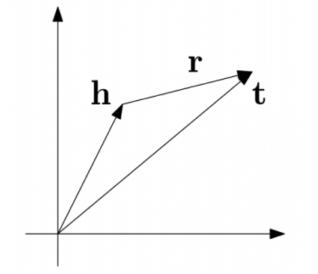受word2vec启发，利用了词向量的平移不变现象。将每个三元组实例 (head，relation，tail) 中的关系 relation 看做从实体 head 到实体 tail 的翻译，通过不断调整h、r和t (head、relation 和 tail 的向量)，使 (h + r) 尽可能与 t 相等，即 h + r ≈ t。数学上表示就是通过约束d(h+l,t)=||(h+r)−t||22≈0$d\left(h+l,t\right)=||\left(h+r\right)-t|{|}_{2}^{2}\approx 0$$d(h+l,t)=||(h+r)-t||_2^2\approx0$来对实体和关系建模，将它们映射到相同的向量空间中。
其损失函数表示如下：

L=∑(h,l,t)∈S∑(h′,l′,t′)∈S′(h,l,t)[γ+d(h+l,t)−d(h′+l′,t′)]+$\mathfrak{L}=\sum _{\left(h,l,t\right)\in S}\sum _{\left({h}^{\mathrm{\prime }},{l}^{\mathrm{\prime }},{t}^{\mathrm{\prime }}\right)\in {S}_{\left(h,l,t\right)}^{\mathrm{\prime }}}\left[\gamma +d\left(h+l,t\right)-d\left({h}^{\mathrm{\prime }}+{l}^{\mathrm{\prime }},{t}^{\mathrm{\prime }}\right){\right]}_{+}$\mathfrak{L} = \sum_{(h,l,t)\in S} \sum_{(h^\prime, l^\prime, t^\prime)\in S_{(h,l,t)}^\prime}[\gamma + d(h+l,t) - d(h^\prime+l^\prime,t^\prime)]_+

其中，[x]+$\left[x{\right]}_{+}$$[x]_+$表示x$x$$x$的正数部分，γ$\gamma$$\gamma$表示margin，S′h,l,t={(h′,l,t|h′∈E)}∪{(h,l,t′|t′∈E)}${S}_{h,l,t}^{\mathrm{\prime }}=\left\{\left({h}^{\mathrm{\prime }},l,t|{h}^{\mathrm{\prime }}\in E\right)\right\}\cup \left\{\left(h,l,{t}^{\mathrm{\prime }}|{t}^{\mathrm{\prime }}\in E\right)\right\}$$S_{h,l,t}^\prime=\lbrace (h^\prime,l,t|h^\prime\in E)\rbrace \cup \lbrace(h,l,t^\prime|t^\prime\in E)\rbrace$

TransH: 将知识嵌入到超平面（Knowledge graph embedding by translating on hyperplanes）

问题：对知识库中的实体关系建模,特别是一对多,多对一,多对多的关系。设计更好的建立负类的办法用于训练。
方案：将实体和关系嵌入到同一的向量空间，但实体在不同关系中有不同的表示。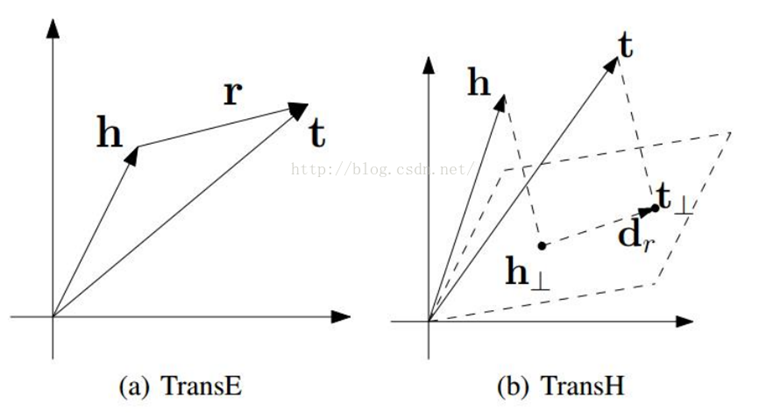在数学表示上面就可以很简单的看出TransH与TransE的区别：TransE中三元组(h,r,t)$\left(h,r,t\right)$$(h,r,t)$需要满足d(h+r,t)=||(h+r)−t||22≈0$d\left(h+r,t\right)=||\left(h+r\right)-t|{|}_{2}^{2}\approx 0$$d(h+r,t)=||(h+r)-t||_2^2\approx0$，而TransH中三元组(h,r,t)$\left(h,r,t\right)$$(h,r,t)$则需要满足d(h+r,t)=||(h−wTrhwr)+dr−(t−wTrtwr)||22≈0$d\left(h+r,t\right)=||\left(h-{w}_{r}^{T}h{w}_{r}\right)+{d}_{r}-\left(t-{w}_{r}^{T}t{w}_{r}\right)|{|}_{2}^{2}\approx 0$$d(h+r,t)=||(h-w_r^Thw_r)+d_r-(t-w_r^Ttw_r)||_2^2\approx0$，其中wr,dr∈Rk${w}_{r},{d}_{r}\in {\mathbb{R}}^{k}$$w_r,d_r\in\mathbb{R}^k$表示关系。

TransR: 实体和关系分开嵌入（Learning Entity and Relation Embeddings for Knowledge Graph Completion）

问题：一个实体是多种属性的综合体，不同关系关注实体的不同属性。直觉上一些相似的实体在实体空间中应该彼此靠近，但是同样地，在一些特定的不同的方面在对应的关系空间中应该彼此远离。
方案：将实体和关系嵌入到不同的空间中，在对应的关系空间中实现翻译。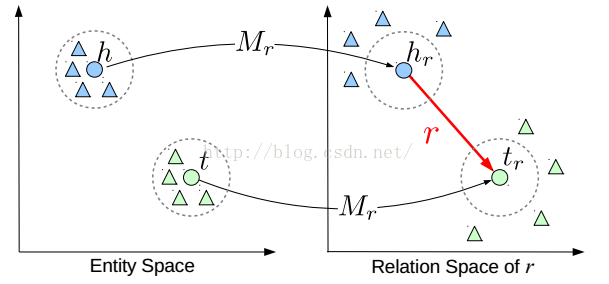TransR在TranE的基础上的改进，在数学上的描述看起来会更加直观：对于每一类关系，不光有一个向量r$r$$r$来描述它自身，还有一个映射矩阵Mr${M}_{r}$$M_r$来描述这个关系所处的关系空间，即对于一个三元组(h,r,t)$\left(h,r,t\right)$$(h,r,t)$，需要满足d(h,r,t)=||hr+r−tr||22=||hMr+r−tMr||22≈0$d\left(h,r,t\right)=||{h}_{r}+r-{t}_{r}|{|}_{2}^{2}=||h{M}_{r}+r-t{M}_{r}|{|}_{2}^{2}\approx 0$$d(h,r,t)=||h_r+r-t_r||_2^2=||hM_r+r-tM_r||_2^2\approx0$。

TransD: 通过动态映射矩阵嵌入（Knowledge graph embedding via dynamic mapping matrix）

问题：TransR过于复杂，在TransR的基础上减少参数。。。
方案：实体和关系映射到不同的空间中，用两个向量表示实体或关系，一个(h,r,t)$\left(h,r,t\right)$$(h,r,t)$表征实体或关系，另一个(hp,rp,tp)$\left({h}_{p},{r}_{p},{t}_{p}\right)$$(h_p,r_p,t_p)$用来构造动态映射矩阵。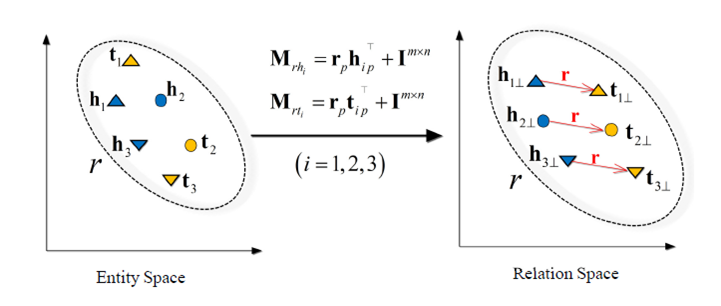TransD在TransR的基础上，将关系的映射矩阵简化为两个向量的积，图中Mrh=rphp+Im∗n${M}_{rh}={r}_{p}{h}_{p}+{I}^{m\ast n}$$M_{rh}=r_ph_p+I^{m*n}$与Mrt=rptp+Im∗n${M}_{rt}={r}_{p}{t}_{p}+{I}^{m\ast n}$$M_{rt}=r_pt_p+I^{m*n}$表示实体h$h$$h$与实体r$r$$r$映射到关系空间的矩阵，那么对于三元组(h,r,t)$\left(h,r,t\right)$$(h,r,t)$，需要满足d(h,r,t)=||Mrhh+r−Mrtt||22≈0$d\left(h,r,t\right)=||{M}_{rh}h+r-{M}_{rt}t|{|}_{2}^{2}\approx 0$$d(h,r,t)=||M_{rh}h+r-M_{rt}t||_2^2\approx0$。

TransA: 自适应的度量函数（An adaptive approach for knowledge graph embedding）

问题：如何解决了translation-based 知识表示方法存在的过于简化损失度量，没有足够能力去度量/表示知识库中实体/关系的多样性和复杂性的问题。
方案：更换度量函数，区别对待向量表示中的各个维度，增加模型表示能力。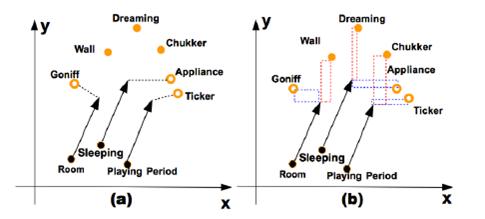光看这张图可能会意义不明，其实模型在TransE的基础上的改进也非常小，简单地说就是给实体/关系的每一个维度都加上了一个权重，增加模型的表示能力。
TransE模型的一般形式为：d(h+l,t)=||(h+r)−t||22 =(h+r−t)T(h+r−t)$d(h+l,t)=||(h+r)-t||_2^2\ = (h+r-t)^T(h+r-t)$
TransA对于每一类关系，给实体/向量空间加上了一个权重矩阵Wr${W}_{r}$$W_r$，然后可以对权重向量做矩阵分解Wr=LTrDrLr${W}_{r}={L}_{r}^{T}{D}_{r}{L}_{r}$$W_r=L_r^TD_rL_r$，最后TransA的数学形式为：d(h+l,t)=(h+r−t)TWr(h+r−t)=(Lr|h+r−t|)TDr(Lr|h+r−t|))$d\left(h+l,t\right)=\left(h+r-t{\right)}^{T}{W}_{r}\left(h+r-t\right)=\left({L}_{r}|h+r-t|{\right)}^{T}{D}_{r}\left({L}_{r}|h+r-t|\right)\right)$$d(h+l,t) = (h+r-t)^T W_r(h+r-t) = (L_r |h+r-t|)^TD_r(L_r|h+r-t|))$。

TranSpare: 自适应稀疏转换矩阵（Knowledge graph completion with adaptive sparse transfer matrix）

关键：针对不同难度的实体间关系，使用不同稀疏程度的矩阵（不同数量的参数）来进行表征，从而防止对复杂关系欠拟合或者对简单关系过拟合；对头尾两种实体采用不同的投影矩阵，解决头尾实体数目不对等的问题。

针对异质性（heterogeneous）

在TransR的基础上，使用可变的稀疏矩阵代替TransR的稠密矩阵：关系连接的实体数量越多，关系越复杂，矩阵约稠密；关系链接的实体数量越少，关系越简单，矩阵约稀疏。
使用参数θr${\theta }_{r}$$\theta_r$描述关系r$r$$r$的复杂程度，使用一个稀疏矩阵Mr(θr)${M}_{r}\left({\theta }_{r}\right)$$M_r(\theta_r)$和一个关系向量r$r$$r$表示一类关系，其中θr=1−(1−θmin)Nr/Nr∗${\theta }_{r}=1-\left(1-{\theta }_{min}\right){N}_{r}/{N}_{{r}^{\ast }}$$\theta_r=1-(1-\theta_{min})N_r/N_{r^*}$，r∗${r}^{\ast }$$r^*$表示连接实体数量最多的关系，Nr∗${N}_{{r}^{\ast }}$$N_{r^*}$为其连接的实体的数量，θmin${\theta }_{min}$$\theta_{min}$为设置的超参，表示关系r∗${r}^{\ast }$$r^*$的稀疏程度。
以此为基础，对于一个关系三元组(h,r,t)$\left(h,r,t\right)$$(h,r,t)$，需要满足的约束为d(h,r,t)=||hp+r−tp||22=||Mr(θr)h+r−Mr(θr)t||22≈0$d\left(h,r,t\right)=||{h}_{p}+r-{t}_{p}|{|}_{2}^{2}=||{M}_{r}\left({\theta }_{r}\right)h+r-{M}_{r}\left({\theta }_{r}\right)t|{|}_{2}^{2}\approx 0$$d(h,r,t)=||h_p+r-t_p||_2^2=||M_r(\theta_r)h+r-M_r(\theta_r)t||_2^2\approx0$。

针对不平衡性（unbalanced）

与上述方法类似，不同点在于对于每个关系三元组(h,r,t)$\left(h,r,t\right)$$(h,r,t)$，头尾实体的映射矩阵为两个不同的稀疏矩阵，其稀疏程度与该关系的头尾实体的数目有关，即头/尾涉及到的实体越多，矩阵约稠密；反之涉及到的实体越少，矩阵越稀疏。
使用参数θhr${\theta }_{r}^{h}$$\theta_r^h$与θtr${\theta }_{r}^{t}$$\theta_r^t$分别描述头尾实体映射矩阵的稠密程度，则θlr=1−(1−θmin)Nlr/Nl∗r∗${\theta }_{r}^{l}=1-\left(1-{\theta }_{min}\right){N}_{r}^{l}/{N}_{{r}^{\ast }}^{{l}^{\ast }}$$\theta_r^l=1-(1-\theta_{min})N_r^l/N_{r^*}^{l^*}$，其中Nlr${N}_{r}^{l}$$N_{r}^{l}$表示关系r$r$$r$在位置l$l$$l$（即头或者尾）上关联的实体数量，Nl∗r∗${N}_{{r}^{\ast }}^{{l}^{\ast }}$$N_{r^*}^{l^*}$则表示关系r∗${r}^{\ast }$$r^*$在位置l∗${l}^{\ast }$$l^*$（即头或者尾）上关联的实体数量最多的关系的数量，对应的设置超参θmin${\theta }_{min}$$\theta_{min}$表示其稀疏程度。
对于一个关系三元组(h,r,t)$\left(h,r,t\right)$$(h,r,t)$，TransSparse需要满足的约束为d(h,r,t)=||hp+r−tp||22=||Mhr(θhr)h+r−Mtr(θtr)t||22≈0$d\left(h,r,t\right)=||{h}_{p}+r-{t}_{p}|{|}_{2}^{2}=||{M}_{r}^{h}\left({\theta }_{r}^{h}\right)h+r-{M}_{r}^{t}\left({\theta }_{r}^{t}\right)t|{|}_{2}^{2}\approx 0$$d(h,r,t)=||h_p+r-t_p||_2^2=||M_r^h(\theta_r^h)h+r-M_r^t(\theta_r^t)t||_2^2\approx0$。

TransG: 高斯混合模型（A Generative Mixture Model for Knowledge Graph Embedding）

问题：解决多关系语义的问题，同一种关系在语义上是不同的，eg, (Atlantics, HasPart, NewYorkBay)与(Table, HasPart, Leg)。
方案：利用贝叶斯非参数高斯混合模型对一个关系生成多个翻译部分，根据三元组的特定语义得到当中的最佳部分。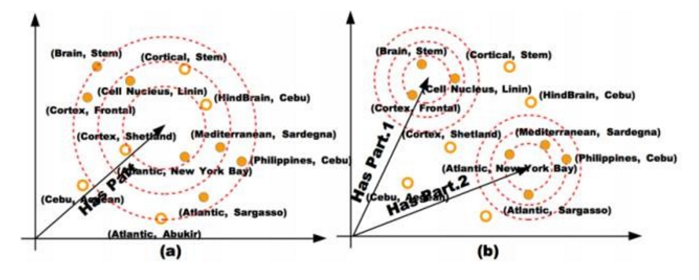考虑到一种关系存在的多语义问题，相当于对关系进行了细化，就是找到关系的隐形含义，最终从细化的结果中选出一个最佳的关系语义。

KG2E: 高斯分步表示实体和关系（Learning to represent knowledge graphs with gaussian embedding）

关键：使用Gaussian Distribution 来表示实体和关系，提出了用Gaussian Distribution的协方差来表示实体和关系的不确定度的新思想，提升了已有模型在link prediction和triplet classification问题上的准确率。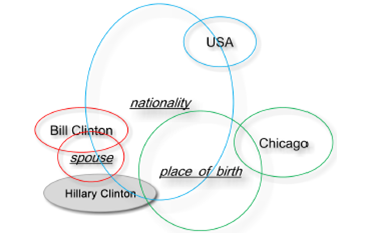ps. 最后两种方法设计到使用高斯混合分步表示实体，后面有时间了再更新。
展开全文TransE 知识图谱 关系三元组
• 第二章 知识表示学习 1. 任务定义、目标和研究意义 　知识表示是知识获取与应用的基础，因此知识表示学习问题，是贯穿知识库 的构建与应用全过程的关键问题。人们通常以网络的形式组织知识库中的知识， 网络中每个...
第二章 知识表示学习
1. 任务定义、目标和研究意义
知识表示是知识获取与应用的基础，因此知识表示学习问题，是贯穿知识库 的构建与应用全过程的关键问题。人们通常以网络的形式组织知识库中的知识， 网络中每个节点代表实体（人名、地名、机构名、概念等），而每条连边则代表 实体间的关系。然而，基于网络形式的知识表示面临诸多挑战性难题，主要包括 如下两个方面：
（1）计算效率问题。基于网络的知识表示形式中，每个实体均用不同的节 点表示。当利用知识库计算实体间的语义或推理关系时，往往需要人们设计专门 的图算法来实现，存在可移植性差的问题。更重要的，基于图的算法计算复杂度 高，可扩展性差，当知识库规模达到一定规模时，就很难较好地满足实时计算的 需求。
（2）数据稀疏问题。与其他类型的大规模数据类似，大规模知识库也遵守 长尾分布，在长尾部分的实体和关系上，面临严重的数据稀疏问题。例如，对于 长尾部分的罕见实体，由于只有极少的知识或路径涉及它们，对这些实体的语义 或推理关系的计算往往准确率极低。
近年来，以深度学习[Bengio, et al., 2009]为代表的表示学习[Bengio, et al., 2013]技术异军突起，在语音识别、图像分析和自然语言处理领域获得广泛关注。 表示学习旨在将研究对象的语义信息表示为稠密低维实值向量。在该低维向量空 间中，两个对象距离越近，则说明其语义相似度越高。 知识表示学习，则是面向知识库中的实体和关系进行表示学习。知识表示学 习实现了对实体和关系的分布式表示，它具有以下主要优点：
（1）显著提升计算效率。知识库的三元组表示实际就是基于独热表示的。 如前所分析的，在这种表示方式下，需要设计专门的图算法计算实体间的语义和 推理关系，计算复杂度高，可扩展性差。而表示学习得到的分布式表示，则能够 高效地实现语义相似度计算等操作，显著提升计算效率。
（2）有效缓解数据稀疏。由于表示学习将对象投影到统一的低维空间中， 使每个对象均对应一个稠密向量，从而有效缓解数据稀疏问题，这主要体现在两 个方面。一方面，每个对象的向量均为稠密有值的，因此可以度量任意对象之间 的语义相似程度。另一方面，将大量对象投影到统一空间的过程，能够将高频对 象的语义信息用于帮助低频对象的语义表示，提高低频对象的语义表示的精确性。
（3）实现异质信息融合。不同来源的异质信息需要融合为整体，才能得到 有效应用。例如，人们构造了大量知识库，这些知识库的构建规范和信息来源均有不同，例如著名的世界知识库有 DBPedia、YAGO、Freebase 等。大量实体和 关系在不同知识库中的名称不同。如何实现多知识库的有机融合，对知识库应用 具有重要意义。通过设计合理的表示学习模型，将不同来源的对象投影到同一个 语义空间中，就能够建立统一的表示空间，实现多知识库的信息融合。此外，当 我们在信息检索或自然语言处理中应用知识库时，往往需要计算查询词、句子、 文档和知识库实体之间的复杂语义关联。由于这些对象的异质性，在往常是棘手 问题。而知识表示学习亦能为此提供统一表示空间，轻而易举实现异质对象之间 的语义关联计算。
综上，由于知识表示学习能够显著提升计算效率，有效缓解数据稀疏，实现 异质信息融合，因此对于知识库的构建、推理和应用具有重要意义，值得广受关 注、深入研究。
2. 研究内容和关键科学问题
知识表示学习是面向知识库中实体和关系的表示学习。通过将实体或关系投 影到低维向量空间，我们能够实现对实体和关系的语义信息的表示，可以高效地 计算实体、关系及其之间的复杂语义关联。这对知识库的构建、推理与应用均有 重要意义。目前，已经在知识图谱补全、关系抽取等任务中取得了瞩目成果。但 是，知识表示学习仍然面临很多挑战。
2.1 复杂关系建模
现有知识表示学习方法无法有效地处理知识图谱中的复杂关系。这里的复杂 关系定义如下。按照知识库中关系两端连接实体的数目，可以将关系划分为 1-1、 1-N、N-1 和 N-N 四种类型。例如 N-1 类型关系指的是，该类型关系中的一个尾 实体会平均对应多个头实体，即我们将 1-N、N-1 和 N-N 称为复杂关系。研究发 现，各种知识获取算法在处理四种类型关系时的性能差异较大，在处理复杂关系 时性能显著降低。如何实现表示学习对复杂关系的建模成为知识表示学习的一个 难点。
2.2 多源信息融合
知识表示学习面临的另外一个重要挑战如何实现多源信息融合。现有的知识 表示学习模型仅利用知识图谱的三元组结构信息进行表示学习，尚有大量与知识 有关的其他信息没有得到有效利用，例如： （1）知识库中的其他信息，如实体和关系的描述信息、类别信息等； （2）知识库外的海量信息，如互联网文本蕴含了大量与知识库实体和关系 有关的信息。 如何充分融合这些多源异质信息，实现知识表示学习，具有重要意义，可改善数据稀疏问题，提高知识表示的区分能力。
2.3 关系路径建模
在知识图谱中，多步的关系路径也能够反映实体之间的语义关系。Lao 等人 曾提出 Path-Constraint Random Walk[Lao, et al., 2010]、Path Ranking Algorithm[Lao, et al., 2010]等算法，利用两实体间的关系路径信息，预测它们的关系，取得显著 效果，说明关系路径蕴含着丰富的信息。如何突破知识表示学习孤立学习每个三 元组的局限性，充分考虑关系路径信息是知识表示学习的关键问题。
3. 技术方法和研究现状
知识表示学习是近年来的研究热点，研究者提出了多种模型，学习知识库中 的实体和关系的表示。本节将主要介绍其中的代表方法。
结构表示[Bordes, et al. 2011]（Structured Embedding，SE）是较早的几个知 识表示方法之一。对于一个事实三元组，SE 将头实体向量和尾实体向量通过关系的两个矩阵投影到关系的对应空间中，然后在该空间中计算两投影向量的距离。 这个距离反映了两个实体在该关系下的语义相关度，它们的距离越小，说明这两 个实体存在这种关系。然而，SE 模型有一个重要缺陷：它对头、尾实体使用两 个不同的矩阵进行投影，协同性较差，往往无法精确刻画两实体与关系之间的语 义联系。
因此，单层神经网络模型[Socher, et al. 2013]（Single Layer Model, SLM） 尝试采用单层神经网络的非线性操作，来减轻 SE 无法协同精确刻画实体与关系 的语义联系的问题。虽然 SLM 是 SE 模型的改进版本，但是它的非线性操作仅 提供了实体和关系之间比较微弱的联系。与此同时，却引入了更加高的计算复杂度。
此外，语义匹配能量模型[Bordes, et al., 2012; Bordes, et al., 2014]（Semantic Matching Energy，SME）提出更复杂的操作，寻找实体和关系之间的语义联系。 在 SME 中，每个实体和关系都用低维向量表示。在此基础上，SME 定义若干投 影矩阵，利用双线性函数来刻画实体与关系的内在联系。
同样利用双线性函数的 还有隐变量模型[Sutskever, et al., 2009; Jenatton, et al., 2012（] Latent Factor Model， LFM），该模型提出利用基于关系的双线性变换，刻画实体和关系之间的二阶联 系。与以往模型相比，LFM 取得巨大突破：通过简单有效的方法刻画了实体和 关系的语义联系，协同性较好，计算复杂度低。
后来的 DISTMULT 模型[Yang, et al., 2015]还探索了 LFM 的简化形式：将关系矩阵设置为对角阵。实验表明，这 种简化不仅极大降低了模型复杂度，模型效果还得到显著提升。
在 LFM 的基础 上，张量神经网络模型[Socher, et al. 2013]（Neural Tensor Network，NTN）进一 步利用关系的双线性变换来刻画实体与关系之间的联系，其基本思想是用双线性 张量取代传统神经网络中的线性变换层，在不同的维度下将头、尾实体向量联系 起来。由于 NTN 引入了张量操作，虽然能够更精确地刻画实体和关系的复杂语义联系，但是计算复杂度非常高，需要大量三元组样例才能得到成分学习。实验 表明，NTN 在大规模稀疏知识图谱上的效果较差。
此外，矩阵分解同样是是得 到低维向量表示的重要途径。因此，也有研究者提出采用矩阵分解进行知识表示 学习。这方面的代表方法是 RESACL模型[Nickel, et al., 2011; Nickel, et al., 2012]。 RESACL 的基本思想与前述 LFM 类似。不同之处在于，RESACL 会优化张量中 的所有位置，包括值为 0 的位置；而 LFM 只会优化知识库中存在的三元组。
最近，Bordes 等人受到词向量空间对于词汇语义与句法关系存在有趣的平移 不变现象的启发，提出了 TransE 模型[Bordes, et al., 2013]，将知识库中的关系看 作实体间的某种平移向量。与以往模型相比，TransE 模型参数较少，计算复杂度 低，却能直接建立实体和关系之间的复杂语义联系。Bordes 等人在 WordNet 和 Freebase 等数据集上进行链接预测等评测任务，实验表明 TransE 的性能较以往 模型有显著提升。特别是在大规模稀疏知识图谱上，TransE 的性能尤其惊人。由 于 TransE 简单有效，自提出以来，有大量研究工作对 TransE 进行扩展和应用。 可以说，TransE 已经成为知识表示学习的代表模型。在 TransE 的基础上，研究 者提出了众多改进模型来解决 TransE 中仍无法处理的问题。
3.1 复杂关系建模
TransE 由于模型简单，在大规模知识图谱上效果明显。但是也由于过于简单， 导致 TransE 在处理前面提到的知识库的复杂关系时捉襟见肘。例如，假如知识 库中有两个三元组，分别是(美国, 总统, 奥巴马)和(美国, 总统, 布什)。这里的 关系“总统”是典型的 1-N 的复杂关系。如果用 TransE 从这两个三元组学习知识 表示，将会使奥巴马和布什的向量变得相同。
为了解决 TransE 模型在处理 1-N、N-1、N-N 复杂关系时的局限性，TransH 模型[Wang, et al., 2014]提出让一个实体在不同的关系下拥有不同的表示。
TransR 模型[Lin, et al., 2015]进一步认为不同的关系拥有不同的语义空间。对每个三元 组，首先应将实体利用矩阵投影到对应的关系空间中，然后再建立从头实体到尾 实体的翻译关系。
针对在知识库中实体的异质性和不平衡性，还有 TransR 模型 中矩阵参数过多的问题，TransD 模型[Ji, et al., 2015]和 TranSparse 模型对 TransR 模型中的投影矩阵进行了进一步的优化。
此外，TransG 模型[Xiao, et al., 2015]和 KG2E 模型[He, et al. 2015]提出了利用高斯分布来表示知识库中的实体 和关系，可以在表示过程中考虑实体和关系本身语义上的不确定性。
可以看到， 在TransE之后，在如何处理复杂关系建模的挑战问题上，提出了TransH、TransR、 TransD、TranSparse、TransG 和 KG2E 等多种模型，从不同角度尝试解决复杂关 系建模问题，可谓百花齐放。在相关数据集合上的实验表明，这些方法均较 TransE 有显著的性能提升，验证了这些方法的有效性。
3.2 多源信息融合
知识表示学习面临的另外一个重要挑战如何实现多源信息融合。现有的知识 表示学习模型如 TransE 等，仅利用知识图谱的三元组结构信息进行表示学习， 尚有大量与知识有关的其他信息没有得到有效利用。如何充分融合这些多源异质 信息，实现知识表示学习，具有重要意义，可以改善数据稀疏问题，提高知识表 示的区分能力。 在融合上述信息进行知识表示学习方面，已经有一些研究工作，但总体来讲 还处于起步状态，这里简单介绍其中几个代表性工作。
考虑实体描述的知识表示学习模型（Description- Embodied Knowledge Representation Learning，DKRL）[Xie, et al., 2016]。DKRL 模型提出在知识表示学习中考虑 Freebase 等知识库中提供的 实体描述文本信息。在文本表示方面，DKRL 考虑了两种模型：
CBOW， 将文本中的词向量简单相加作为文本表示；
卷积神经网络，能够考虑文本 中的词序信息。
DKRL 的优势在于，除了能够提升实体表示的区分能力外，还能 实现对新实体的表示。当新出现一个未曾在知识库中的实体时，DKRL 可以根据 它的简短描述产生它的实体表示，用于知识图谱补全等任务。这对于不断扩充知 识图谱具有重要意义。 此外，Wang 等人提出在表示学习中考虑文本数据，利用 word2vec 学习维基 百科正文中的词表示，利用 TransE 学习知识库中的知识表示。然后利用维基百 科正文中的链接信息（锚文本与实体的对应关系），让文本中实体对应的词表示 与知识库中的实体表示尽可能接近，从而实现文本与知识库融合的表示学习。 Zhong 等人还将类似的想法用于融合实体描述信息[Zhong, et al., 2015]。 已有工作表明，多源信息融合能够有效提升知识表示的性能，特别是可以有 效处理新实体的表示问题。但是，也可以看出，多源信息融合的知识表示学习仍 处于非常起步的阶段，相关工作较少，考虑的信息源非常有限，有大量的信息（如 音频、图片、视频等）未被考虑，具有广阔的研究前景。
3.3 关系路径建模
在知识图谱中，多步的关系路径也能够反映实体之间的语义关系。为了突破 TransE 等模型孤立学习每个三元组的局限性，Lin 等人提出考虑关系路径的表示 学习方法，以 TransE 作为扩展基础，提出 Path-based TransE（PTransE）模型[Lin, et al., 2015]。几乎同时，也有其他研究团队在知识表示学习中成功考虑了关系路 径的建模[Alberto, et al., 2015]。关系路径的表示学习也被用来进行基于知识库的 自动问答[Gu, et al., 2015]。 PTransE 等研究的实验表明，考虑关系路径能够极大提升知识表示学习的区分性，提高在知识图谱补全等任务上的性能。关系路径建模工作还比较初步，在关系路径的可靠性计算，关系路径的语义组合操作等方面，还有很多细致的考察 工作需要完成。
4. 技术展望与发展趋势
近年来知识表示学习已经崭露头角，在很多任务上展现了巨大的应用潜力。 对于 TransE 等模型面临的挑战，也已经提出了很多改进方案。然而，知识表示 学习距离真正实用还很远，本节将对知识表示学习的未来方向进行展望。
面向不同知识类型的知识表示学习 已有工作将知识库的关系划分为 1-1、1-N、N-1 和 N-N 四类，这种关系类 型划分略显粗糙，无法直观地解释知识的本质类型特点。近期发表在 Science 等 权威期刊的认知科学研究成果[Kemp, et al., 2009; Tenenbaum, et al., 2011] 总结认 为，人类知识包括以下几种结构：
（1）树状关系，表示实体间的层次分类关系；
（2）二维网格关系，表示现实世界的空间信息；
（3）单维顺序关系，表示实体 间的偏序关系；
（4）有向网络关系，表示实体间的关联或因果关系。
认知科学对 人类知识类型的总结，有助于对知识图谱中知识类型的划分和处理。未来有必要 结合人工智能和认知科学的最新研究成果，有针对性地设计知识类型划分标准， 开展面向不同复杂关系类型的知识表示学习研究。
多源信息融合的知识表示学习
在多源信息融合的知识表示学习方面，相关工作还比较有限，主要是考虑实 体描述的知识表示学习模型，以及文本与知识库融合的知识表示学习，这些模型 无论是信息来源，还是融合手段都非常有限。我们认为在多源信息融合的知识表 示学习方面，我们还可以对下列方面进行探索：
（1）融合知识库中实体和关系的 其他信息，知识库中拥有关于实体和关系的丰富信息，如描述文本、层次类型等。 有机融合这些信息，将显著提升知识表示学习的表示能力；
（2）融合互联网文本、 图像、音频、视频信息，互联网海量文本、音频、视频数据是知识库的重要知识 来源，有效地利用这些信息进行知识表示可以极大地提升现有知识表示方法的表 示能力；
（3）融合多知识库信息，人们利用不同的信息源构建了不同的知识库。 如何对多知识库信息进行融合表示，对于建立统一的大规模知识库意义重大。
考虑复杂推理模式的知识表示学习
考虑关系路径的知识表示学习，实际上是充分利用了两实体间的关系和关系 路径之间的推理模式，来为表示学习模型提供更精确的约束信息。例如，根据三 元组（康熙，父亲，雍正）和（雍正，父亲，乾隆）构成的“康熙”和“乾隆”之间 “父亲+父亲”的关系路径，再结合三元组（康熙，祖父，乾隆），PTransE 实际上 额外提供了“父亲+父亲=祖父”的推理模式，从而提升知识表示的精确性。
实际上，关系路径只是复杂推理模式中的一种特殊形式，它要求头实体和尾实体必须保持不变。但实际上，知识库中还有其他形式的推理模式，例如三元组 （美国，总统，奥巴马）和（奥巴马，是，美国人）之间就存在着推理关系，但 是两者的头、尾实体并不完全一致。如果能将这些复杂推理模式考虑到知识表示 学习中，将能更进一步提升知识表示的性能。 在该问题中，如何总结和表示这些复杂推理模式，是关键难题。目前来看， 一阶逻辑（First-Order Logic，FOL）是对复杂推理模式的较佳表示方案，未来我 们需要探索一阶逻辑的分布式表示，及其融合到知识表示学习中的技术方案。
面向大规模知识库的在线学习和快速学习 大规模知识库稀疏性很强。初步实验表明，已有表示学习模型在大规模知识 库上性能堪忧，特别是对低频实体和关系的表示效果较差。而且知识库规模不断 扩大，我们需要设计高效的在线学习方案。除了充分融合多源信息降低稀疏性之 外，我们还可以探索如何优化表示学习的方式，借鉴课程学习和迁移学习等算法 思想，进一步改善知识表示的效果。
基于知识分布式表示的应用 知识表示学习还处于起步阶段，在知识获取、融合和推理等方向均有广阔的 应用空间。我们需要在若干重要任务上探索和验证知识表示学习的有效性。例如， 关系抽取任务如果能够基于知识表示学习有效利用知识库信息，将能够极大提升 抽取性能和覆盖面。再如，我们可以充分利用表示学习在信息融合上的优势，实 现跨领域和跨语言的知识库融合。目前，知识分布式表示的作用已经在信息抽取、 自动问答、信息检索、推荐系统中得到初步验证，未来还需在更多任务上进行更 加深入的探索。

转载于:https://www.cnblogs.com/the-wolf-sky/p/11067906.html
展开全文• 最近在看知识图谱知识表示学习(Representation Learning) 本文转载于https://chuansongme.com/n/1006269942667 引   本期PaperWeekly的主题是基于翻译模型(Trans系列)的知识表示学习，主要用来解决知识表示和...
• 本期论文清单来自清华大学博士生韩旭和北师大本科生曹书林，涵盖了近年知识表示学习方向的重要论文。[ 综述类 ]■ 论文 | Representation Learning: A Review and New Perspectives■ 链接 | ...
• 知识图谱最新权威综述论文解读：开篇部分 今天分享一个自己从数据爬取到d3可视化的中式菜谱知识图谱可视化系统的搭建流程。 Github项目地址：https://github.com/ngl567/CookBook-KG 访问可视化系统GithubPage地址：...自然语言处理
• Word Representation1. one-hot representation ...互相正交，难以表示词语之间的相似性 2. distributed representation(词向量) 稠密、实值、低维的向量 便于Deep Learning 相似度用距离表示 word2vec ki知识图谱 词向量 TransE
• dataset #relation #entity # triple(train/valild/test) WN11 11 38696 112581 &amp;nbsp; &amp;nbsp; 2609 &amp;nbsp;&amp;nbsp; 10544 WN18 18 40943 141442 &amp;...13
• KG Embedding学习，只涉及TransE、TransH、TransR、TransDNLP TransE
• 清华大学自然语言处理实验室近日发布了 OpenKE 平台，整合了 TransE、TransH、TransR、TransD、RESCAL、DistMult、HolE、ComplEx 等算法的统一接口高效实现，以及面向 WikiData 和 Freebase 预训练知识表示模型。...
• 刘知远：今天跟大家分享的题目叫"知识表示学习及其应用"。 大概在二三十年前，我们早就面临所谓的数据过载的问题,当时就有一个专门的领域叫信息检索，研究如何在大数据里面快速地获取相关的信息。 搜索引擎是一个...
• 在 OpenKE 中，我们提供了快速和稳定的工具包，包括最流行的知识表示学习（knowledge representation learning，KRL）方法。该框架具有容易拓展和便于设计新的知识表示学习模型的特点。 该框架有如下特征：...
• ## 知识图谱表示学习

千次阅读 2018-06-26 10:49:03
知识图谱是一种精细化的异构网络, 所以对其节点与边的表示学习也是一个热门的问题. 这里的学习依旧是得到它们的低维稠密向量. 参考 论文transr
•知识图谱
• 知识表示学习研究进展》 基于翻译模型（Trans系列）的知识表示学习 然后是清华大学开源OpenKE：知识表示学习平台 “表示学习旨在将研究对象的语义信息表示为稠密低维实值向量，知识表示学习主要是面向知识图谱中...
• 作者丨谢若冰单位丨腾讯微信搜索应用部研究方向丨知识表示学习知识图谱被广泛地用来描述世界上的实体和实体之间的关系，一般使用三元组（h,r,t）（head entity, relation, trail entity）的形式来存储知识，其中蕴含...
•transe
• 知识表示学习的基本思想就是把知识图谱中的三元组（h,r,t) （原文中是（h,l,t))表示为低维稠密向量的形式，传统的图的网络结构存在缺陷，比如说数据稀疏问题，图遍历算法复杂度高等问题。 TransE是Trans系列论文最早...TransE TransH TransR
• 传统的知识图谱的KR，从逻辑和推理讲起，有一阶逻辑(first-order logic)和描述逻辑(description logic)，后来又有逻辑程序(logic program)和生成规则（Production Rule）。知识表现的数据结构，一般来说是那些“复杂...
• 传统的知识图谱表示方法是采用OWL、RDF等本体语言进行描述；随着深度学习的发展与应用，我们期望采用一种更为简单的方式表示，那就是【向量】，采用向量形式可以方便我们进行之后的各种工作，比如：推理，所以，我们...TransE
• ## 表示学习

千次阅读 2018-05-15 17:40:44
尽管可以使用特定的领域知识来帮助设计表示，但也可以使用通用的先验知识进行学习，而对AI的追求则激励设计更强大的表示学习算法来实现这样的先验知识。 本文回顾了近期在无监督特征学习和深度学习方面的工作，包括...深度学习 无监督学习 神经网络
• 1 代码来源 本代码来源于github项目地址，项目实现了TransE算法。下面结合项目代码，对TransE算法原理及实现进行详细说明。 ...我们现在有很多很多的知识库数据knowledge bases (KBs)，比如Fre...
• 网络结构 单层前馈网络、多层前馈网络、递归网络。 知识表示 知识是人或奇迹存储起来以备使用的信息或模型，用来对外部世界作出解释、预测、和适当反应。 知识表示的规则神经网络
• 鉴于知识图谱的研究越来越多，所以在组会主讲上介绍了知识图谱表示学习的Trans系列方法，以下仅是本人对于此类方法的理解，请批评指正。Trans系列方法的源码均为公开代码，可以自行在GitHub中搜索。 背景介绍 谷歌...
• 系列算法 TransE：同一语义空间表示，向量加法 TransH：让一个实体在不同关系下有不同的表示 TransR：认为不同的关系拥有不同的语义空间（实体向关系...TransA：损失函数中距离度量改用马氏距离，并为每一维的学习...
• 作者：张文，浙江大学在读博士，研究方向为知识图谱的表示学习，推理和可解释。本文是我们与苏黎世大学以及阿里巴巴合作的工作，发表于WWW2019，这篇工作将知识图谱推理的两种...
• 知识表示：将人类知识形式化或者模型化。 知识表示方法：符号表示法、连接机制表示法。 机器感知：使机器（计算机）具有类似于人的感知能力。以机器视觉(machine vision)与机器听觉为主。 机器思维：对通过感知得来...人工智能 自然语言处理
• 互联网时代，人类在与自然和社会的交互中生产了异常庞大的数据，这些数据中包含了大量描述自然界和人类社会客观规律有用信息。...编辑 | 小Dream哥 1 什么是知识表示知识表示是知识图谱中非常重要的概念，知......